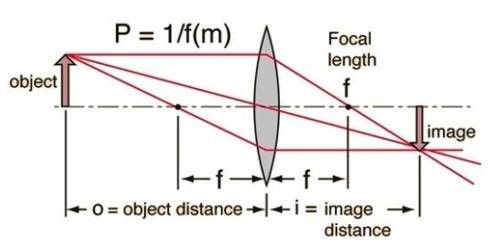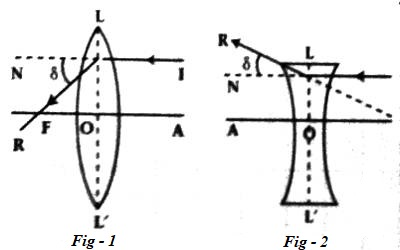# Power of a Lens

Power of a lens

Definition: The power of a lens means its power of convergence in case of a convex lens or its power of divergence in case of a concave lens [Fig. 1 and 2]. If a lens can converge or diverge a beam of light more, then its power is more and if it can converge or diverge less then its power is less. So, the power of a lens is determined by the angle of deviation δ of the refracted rays of the incident rays parallel to the principal axis of the lens. Greater the value of δ for a lens, the more will be the power of that lens. Again in case of the lens of shorter focal length, ft the rays converges or diverge at a point nearer to the lens then that Lens is said to have a greater power. The convergence or divergence, as the case may be, produced by a lens will be greater, the shorter the focal length. So, the reciprocal of the focal length is taken as the measure of the power of a lens.Suppose the focal length of a lens = ƒ; so its power, P = 1/ƒ.

Unit of the power of the lens: Power of lens is a measurable quantity. So it has a unit. The unit of power of the lens is Diopter. In short, it is denoted by D. The reciprocal of the focal length expressed in meter gives the power of the lens in Diopter.

Suppose, the focal length of a lens is ƒ(m). So its power is,

P = 1/ƒ(m) Diopter

So, P = 1/ƒ(m) D … … … (6.12)

The power of a convex lens is a positive quantity whereas the power of a concave lens is a negative quantity.

‘The power of a spectacle is + 4 Diopter’ — what does it mean?

Here, P = + 4 Diopter

So, ƒ = + ¼ m = 0.25 m.

So, the meaning of the power of the spectacle + 4 Diopter is that the lens used in it is a convex lens whose focal length is 0.25 m.

Again the power of a lens is -2D means that the lens is a concave lens and its focal length, ƒ = – ½ m = – 0.5 m.